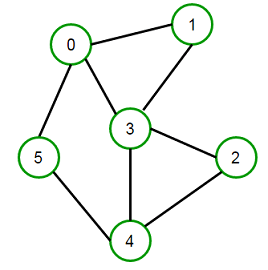# 2-Vertex Connectivity in the graph

Given a undirected connected graph, check if the graph is 2-vertex connected or not.

A connected graph G is said to be 2-vertex-connected (or 2-connected) if it has more than 2 vertices and remains connected on removal of any vertices. Any such vertex whose removal will disconnected the graph is called Articulation point.

For example, Consider below connected graph on the left, if we remove vertex 3 or vertex 4 from the graph, the graph will be disconnected in two connected component. So we can say that 3 and 4 are the Articulation points and graph is not 2-vertex connected. If we add edges (0 -> 1), (0 -> 5) and (2 -> 4) in the graph, it will become 2-vertex connected (check graph on the right).We can find Articulation points in a graph using DFS. We can say that the graph is 2-vertex connected if and only if for every vertex u in the graph, there is at-least one back-edge that is going out of subtree rooted at u to some ancestor of u. When we say subtree rooted at u, we mean all u’s descendants (excluding vertex u). In other words, when we backtrack from a vertex u, we need to ensure that there is some back-edge from some descendant (children) of u to some ancestor (parent or above) of u. There is an exception to this rule for the root of the tree. If the root has more than one children, then it is an articulation point, otherwise not.

Please note that vertex u and v might be confusing to readers in this post. So please read the post carefully and remember that for an edge u -> v, to check weather or not u is articulation point or not we run DFS on v, not on u.

How should we modify DFS so that we can check if there is a back-edge going out of every sub-tree rooted at u?

We can modify DFS such that DFS(v) returns the smallest arrival time to which there is an back edge from the sub tree rooted at v (including v) to some ancestor of vertex u. For example, let arrival(v) be the arrival time of vertex v in the DFS. Then if there is a back out of the sub tree rooted at v, it’s to something visited before v & therefore with a smaller arrival value. Remember for a back edge u -> v in a graph,

`arrival[u] > arrival[v]`

Suppose there are four edges going out of sub-tree rooted at v to vertex a, b, c and d and with arrival time A(a), A(b), A(c) and A(d) respectively. We look at their four arrival times & consider the smallest among them keeping in mind that the back-edge goes to an ancestor of vertex u (and not to vertex u itself) and that will be the value returned by DFS(v). But before returning, check if min(A(a), A(b), A(c), A(d)) is more than the A(u). If yes, then that means that no back-edge is going out of the sub tree rooted at v and u is an articulation point.

Time complexity of above solution will be O(n + m) where n is number of vertices and m is number of edges in the graph.

Applications – To check if the graph is biconnected or not. A biconnected graph is a connected graph on two or more vertices having no articulation vertices.(1 votes, average: 5.00 out of 5)Loading...

Please use our online compiler to post code in comments. To contribute, get in touch with us.
Like us? Please spread the word and help us grow. Happy coding 🙂